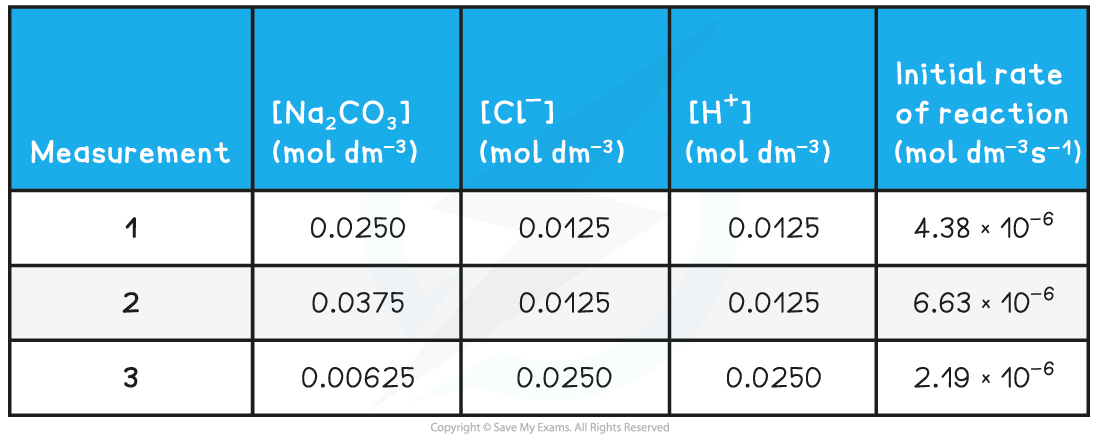# AQA A Level Chemistry复习笔记5.2.3 Rate Equation Calculations

### Rate Equation Calculations

• The rate constant (k) of a reaction can be calculated using the initial rates and the rate equation

#### Calculating the rate constant from the initial rate

• The reaction of sodium carbonate with chloride ions (from hydrochloric acid) to form sodium chloride will be used as an example to calculate the rate constant from the initial rate and initial concentrations
• The reaction and rate equation are as follows:

Na2CO3 (s) + 2Cl- (aq) + 2H+ (aq) → 2NaCl (aq) + CO2 (g) + H2O (l)• The progress of the reaction can be followed by measuring the initial rates of the reaction using various initial concentrations of each reactant

Experimental results of concentrations & initial rates table• To find the rate constant (k):
• Substitute the values of one of the experiments to find k (for example measurement 1)• The values of measurement 2 or 3 could also have been used to find k
• They all give the same result of 1.40 x 10-2

#### Calculating Units

• When you are asked to calculate the rate constant, k, for a reaction you must also be able to deduce the units
• This is done by replacing the values in the rearranged rate equation with the units of that value
• The units can then be combined or cancelled as required
• For example, to calculate the units for the above reaction: